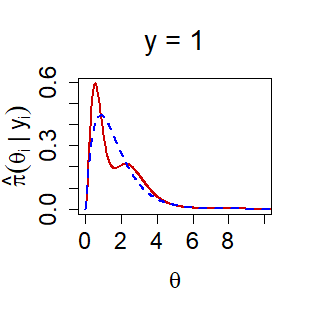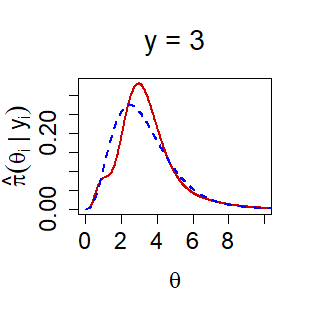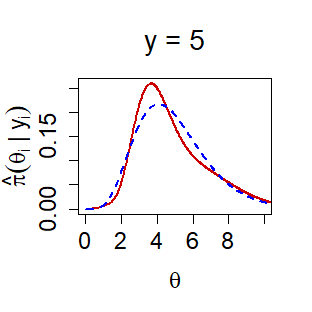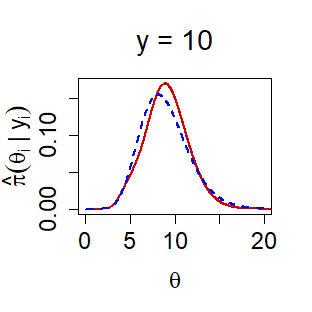# Bayesian Modeling via Frequentist Goodness-of-Fit

## I. Illustration using rat tumor data (Binomial Family)

The rat tumor data consists of observations of endometrial stromal polyp incidence in $$k=70$$ groups of rats. For each group, $$y_i$$ is the number of rats with polyps and $$n_i$$ is the total number of rats in the experiment. Here we describe the analysis of rat tumor data using Bayes-$${\rm DS}(G,m)$$ modeling.

### Pre-Inferential Modeling

Step 1. We begin by finding the starting parameter values for $$g \sim Beta(\alpha, \beta)$$ by MLE:

library(BayesGOF)
set.seed(8697)
data(rat)
###Use MLE to determine starting values
rat.start <- gMLE.bb(rat$y, rat$n)$estimate We use our starting parameter values to run the main function: rat.ds <- DS.prior(rat, max.m = 6, rat.start, family = "Binomial") Step 2. We display the U-function to quantify and characterize the uncertainty of the a priori selected $$g$$: plot(rat.ds, plot.type = "Ufunc")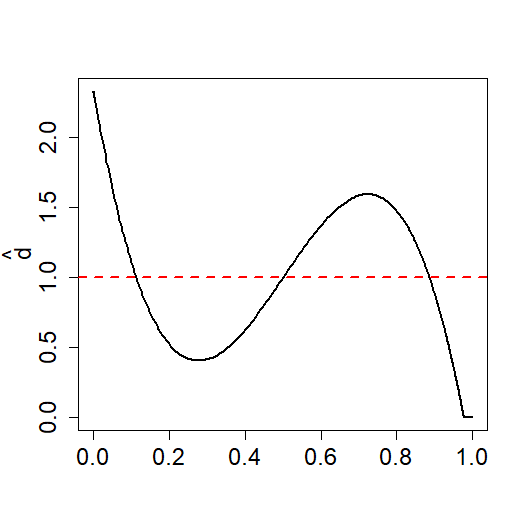The deviations from the uniform distribution (the red dashed line) indicate that our initial selection for $$g$$, $$\text{Beta}(\alpha = 2.3,\beta = 14.1)$$, is incompatible with the observed data and requires repair; the data show that there are, in fact, two different groups of incidence in the rats. Step 3a. Extract the parameters for the corrected prior $$\widehat{\pi}$$: rat.ds ##$g.par
##     alpha      beta
##  2.304768 14.079707
##
## $LP.coef ## LP1 LP2 LP3 ## 0.0000000 0.0000000 -0.5040361 Therefore, the DS prior $$\widehat{\pi}$$ given $$g$$ is: $\widehat{\pi}(\theta) = g(\theta; \alpha,\beta)\Big[1 - 0.52T_3(\theta;G) \Big]$ Step 3b. We can now plot the estimated DS prior $$\widehat{\pi}$$ along with the original parametric $$g$$: plot(rat.ds, plot.type = "DSg", main = "DS vs g: Rat")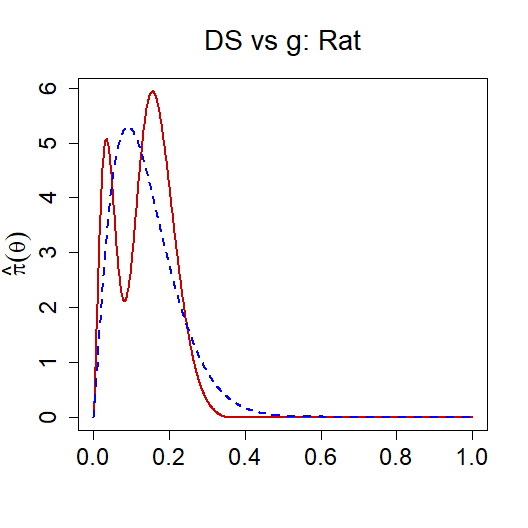### MacroInference Step 4. Here we are interested in the overall macro-level inference by combining the $$k=70$$ parallel studies. The group-specific modes along with their standard errors are computed as follows: rat.macro.md <- DS.macro.inf(rat.ds, num.modes = 2 , iters = 15, method = "mode")  rat.macro.md ## 1SD Lower Limit Mode 1SD Upper Limit ## [1,] 0.0136 0.0340 0.0544 ## [2,] 0.1431 0.1562 0.1692 plot(rat.macro.md, main = "MacroInference: Rat")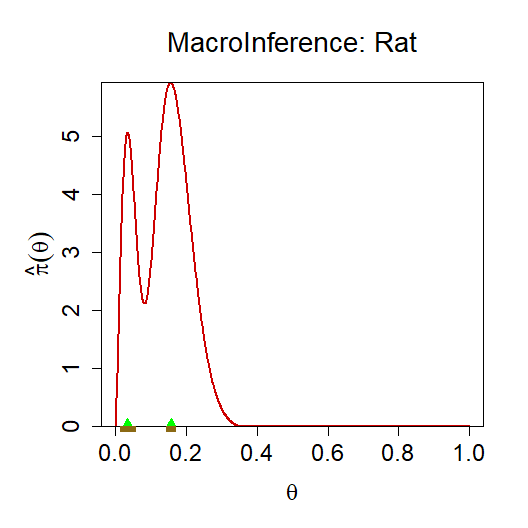### MicroInference Step 5. Given an additional study $$\theta_{71}$$ where $$y_{71} = 4$$ and $$n_{71} = 14$$, the goal is to estimate the probability of a tumor for this new clinical study. The following code performs the desired microinference to find the posterior distribution $$\pi_{LP}(\theta_{71}|y_{71},n_{71})$$ along with its mean and mode: rat.y71.micro <- DS.micro.inf(rat.ds, y.0 = 4, n.0 = 14) rat.y71.micro ## Posterior Mean = 0.1904 ## Posterior Mode = 0.1832 ## Use plot(x) to generate posterior plot plot(rat.y71.micro, main = "Rat (4,14)")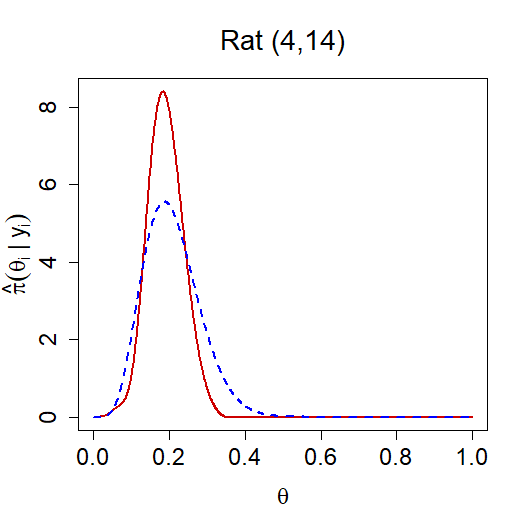### Finite Bayes The previous microinferece step ignores the randomness in the estimated hyperparameters (see step 3a). To take into account this extra variability (of the hyperparameter estimates in our DS(G,m) model) we perform finite Bayes adjustment to our microinference for $$\theta_{71}$$. We also report the 90% Bayesian credible interval based on the finite-sample “corrected” posterior of $$\theta_{71}$$. rat.y71.FB <- DS.Finite.Bayes(rat.ds, y.0 = 4, n.0 = 14, iters = 15) rat.y71.FB ## Posterior Mean = 0.193 ## Posterior Mode = 0.1742 ## Lower Bound Credible Interval = 0.1001 ## Upper Bound Credible Interval = 0.3033 ## Use plot(x) to generate finite Bayes plot plot(rat.y71.FB, xlim = c(0,.5), ylim = c(0, 9)) legend("topright", c("DS Post", "DS Finite Bayes Post", "Parametric Post"), col = c("red", "darkgreen", "blue"), lty = c("solid","solid","dashed"), cex = 1)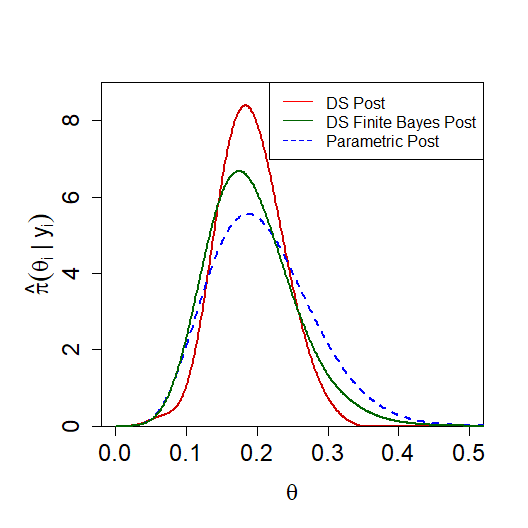## II. Comparison of $$\mathcal{L}^2$$ and maximum entropy representations using galaxy data (Normal Family) This demonstration will compare the results using the default $$\mathcal{L}^2$$ representation of the estimated DS prior $$\widehat{\pi}$$ to the maximum entropy $$\breve{\pi}$$ representation. We will use the galaxy data, which consists of $$k=324$$ observed rotation velocities $$y_i$$ and their uncertainties of Low Surface Brightness (LSB) galaxies. Step 1. We begin by finding the starting parameter values for $$g \sim Normal(\mu, \tau^2)$$ by MLE: data(galaxy) gal.start <- gMLE.nn(galaxy$y, galaxy$se, method = "DL")$estimate

Step 2. Use our starting parameters to run the main function for two disinct cases:

• LP.type = “L2” estimates the DS prior in the $$\mathcal{L}^2$$ representation. This is the default case.

• LP.type = “MaxEnt” estimates the DS prior in terms of the maximum entropy representation.

gal.ds.L2 <- DS.prior(galaxy[,c(1,2)], max.m = 6, g.par = gal.start, family = "Normal", LP.type = "L2")
gal.ds.ME <- DS.prior(galaxy[, c(1,2)], max.m = 6, g.par = gal.start, family = "Normal", LP.type = "MaxEnt")

Step 3. We plot the U-functions and DS priors for both cases for comparison.

plot(gal.ds.L2, plot.type = "Ufunc", main = "L2 U-function")
plot(gal.ds.L2, plot.type = "DSg", main = "L2 DS vs g")
plot(gal.ds.ME, plot.type = "Ufunc", main = "MaxEnt U-function")
plot(gal.ds.ME, plot.type = "DSg", main = "MaxEnt DS vs g")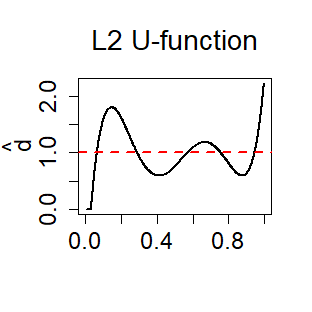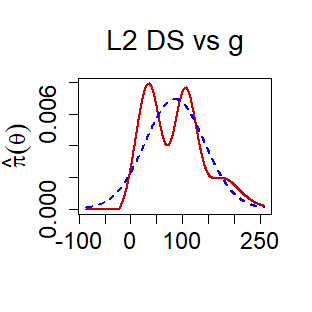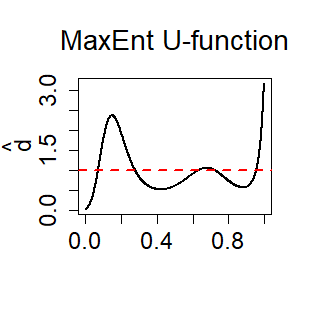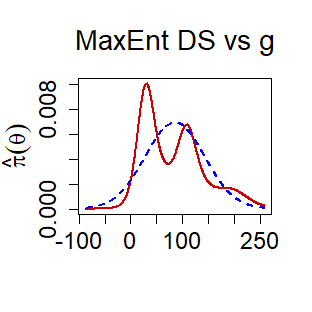The U-function plots maintain a similar structure, but show some slight differences with respect to the two peaks. The maximum entropy representation shows the first peak as more narrow, while the second peak is not as pronounced as the $$\mathcal{L^2}$$ representation. We can see how those slight changes in the U-functions influence the resulting DS-prior in the “DS vs g” plots. The maximum entropy representation has a more significant first peak, smaller second peak, and smoother tails.

Step 4. Extract the parameters for the corrected priors: $$\widehat{\pi}$$ and $$\breve{\pi}$$:

gal.ds.L2
## $g.par ## mu tau^2 ## 85.51316 3304.41491 ## ##$LP.coef
##        LP1        LP2        LP3        LP4        LP5
##  0.0000000  0.0000000  0.2131113 -0.1967060  0.4069104
gal.ds.ME
## $g.par ## mu tau^2 ## 85.51316 3304.41491 ## ##$LP.coef
##    LP(ME)0    LP(ME)1    LP(ME)2    LP(ME)3    LP(ME)4    LP(ME)5
## -0.1529718  0.0000000  0.0000000  0.2553139 -0.2765342  0.4560368

The DS prior in its $$\mathcal{L}^2$$ representation is: $\widehat{\pi}(\theta) = g(\theta; \mu, \tau^2)\Big[1 + 0.21T_3(\theta;G)-0.20T_4(\theta;G)+0.41T_5(\theta;G)\Big]$.

The DS prior in its maximum entropy representation is: $\breve{\pi}(\theta) = g(\theta; \mu, \tau^2)\exp\Big[-0.15 + 0.26T_3(\theta;G)-0.28T_4(\theta;G)+0.46T_5(\theta;G)\Big]$

## III. Illustration using arsenic data (Normal Family)

For this example, we will focus on the macroinference for the arsenic data set. The arsenic data set details the measurements of the level of arsenic in oyster tissue from $$k=28$$ laboratories. We will use the maximum entropy representation for this example.

### Pre-Inferential Modeling

Step 1. We begin by finding the starting parameter values for $$g \sim Normal(\mu, \tau^2)$$ by MLE:

data(arsenic)
arsn.start <- gMLE.nn(arsenic$y, arsenic$se, method = "DL")$estimate We use our starting parameter values to run the main DS.prior function: arsn.ds <- DS.prior(arsenic, max.m = 4, arsn.start, family = "Normal", LP.type = "MaxEnt") Step 2. We display the U-function to quantify and characterize the uncertainty of the a priori selected $$g$$: plot(arsn.ds, plot.type = "Ufunc")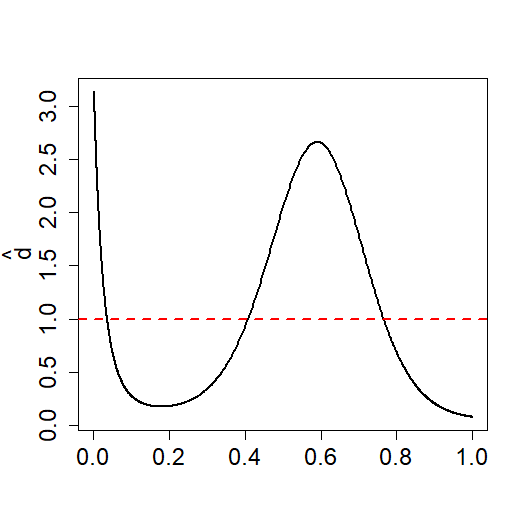Step 3. We now extract the parameters for the corrected prior $$\breve{\pi}$$ and plot it, along with the original $$g$$: arsn.ds ##$g.par
##        mu     tau^2
## 13.220522  3.407165
##
## $LP.coef ## LP(ME)0 LP(ME)1 LP(ME)2 LP(ME)3 LP(ME)4 ## -0.4780663 0.0000000 -0.6503293 -0.7091040 0.4174869 The resulting equation for $$\breve{\pi}(\theta)$$ is: $\breve{\pi}(\theta) = g(\theta; \mu, \tau^2)\exp\Big[-0.48 - 0.65T_2(\theta;G)-0.71T_3(\theta;G)+0.42T_4(\theta;G)\Big]$ plot(arsn.ds, plot.type = "DSg", main = "DS vs g: Arsenic")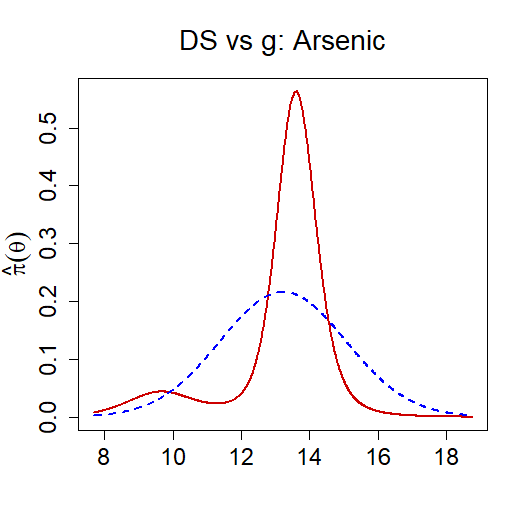### MacroInference Step 4. We now execute the macroinference to find a global estimate to summarize the $$k = 28$$ studies. arsn.macro <- DS.macro.inf(arsn.ds, num.modes = 2, iters = 20, method = "mode") arsn.macro ## 1SD Lower Limit Mode 1SD Upper Limit ## [1,] 8.4138 9.6674 10.9210 ## [2,] 13.3589 13.6030 13.8471 Based on our results, we find two significant modes. Therefore, the prior shows structured heterogeneity and requires both modes to describe the distribution and its two groups. In this case, though, we are looking to estimate the consensus value of the measurand and its uncertainty. Therefore, we would select the dominant mode, which requires the macroinference results: #plot(arsn.macro, main = "MacroInference: Arsenic Data") par(mar=c(5,5.2,4,2)+0.3) #changes left margin to make large labels fit plot(arsn.macro$prior.fit$theta.vals, arsn.macro$prior.fit$ds.prior, type = "l", xlim = c(8,18.5), lwd = 2, col = "red3", xlab = expression(theta), ylab = "", font.main = 1, cex.lab=1.45, cex.axis=1.5, cex.main= 1.75, cex.sub=1.5, main = "MacroInference: Arsenic Data") title(ylab = expression(paste(hat(pi)(theta))), line = 2.3, cex.lab=1.45) points(arsn.macro$model.modes,-.02, col = "green", pch = 17, cex = 1.5)
axis(1, at=seq(arsn.macro$model.modes-arsn.macro$mode.sd,
arsn.macro$model.modes+arsn.macro$mode.sd,
length= (3-2) * 20),tick=TRUE, col="goldenrod4", labels = F, tck=-0.015)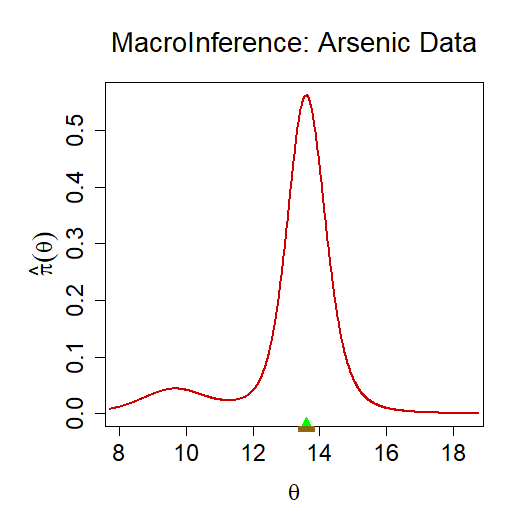The plot shows the dominant mode and our consensus value for the measurand is 13.6 with a standard error (using smooth bootstrap) of 0.25. This mode is resistant to any extreme low measurements and achieves a slightly more accurate result than the standard PEB estimate ($$13.22 \pm 0.25$$).

## IV. Illustration using child illness data (Poisson Family)

The next example will conduct microinference on the child illness data. The child illness data comes from a study where researchers followed $$k=602$$ pre-school children in north-east Thailand, recording the number of times ($$y_i$$) a child became sick during every 2-week period for over three years. In particular, we want to compare posterior distributions for the number of children who became sick 1, 3, 5, and 10 times during a two week period. We will use the $$\mathcal{L}^2$$ representation for this example.

### Pre-Inferential Modeling

Step 1. We begin by finding the starting parameter values for $$g \sim Gamma(\alpha, \beta)$$ by MLE:

data(ChildIll)
child.start <- gMLE.pg(ChildIll)

We use our starting parameter values to run the main DS.prior function for the Poisson family:

child.ds <- DS.prior(ChildIll, max.m = 8, child.start, family = "Poisson")

Step 2. We display the U-function to quantify and characterize the uncertainty of the selected $$g$$:

plot(child.ds, plot.type = "Ufunc")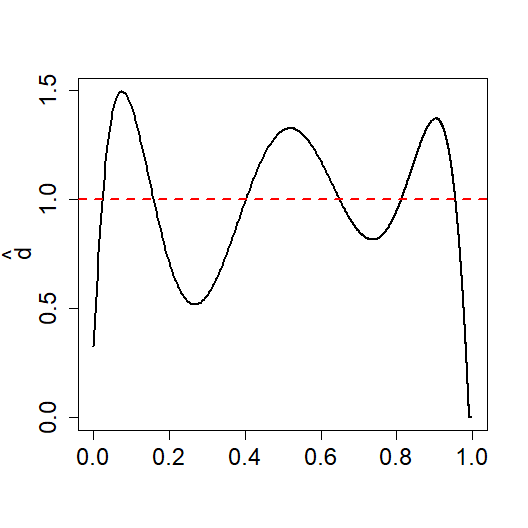Step 3. We now extract the parameters for the corrected prior $$\widehat{\pi}$$:

child.ds
## $g.par ## alpha beta ## 1.060878 4.193337 ## ##$LP.coef
##        LP1        LP2        LP3        LP4        LP5        LP6
##  0.0000000  0.0000000 -0.1259159  0.0000000  0.0000000 -0.2797667

The DS prior $$\widehat{\pi}$$ given $$g$$ is: $\widehat{\pi}(\theta) = g(\theta; \alpha,\beta)\Big[1 - 0.13T_3(\theta;G) - 0.28T_6(\theta;G) \Big].$ We can plot $$\widehat{\pi}$$, along with $$g$$:

plot(child.ds, plot.type = "DSg", main = "DS vs. g: Child Illness Data")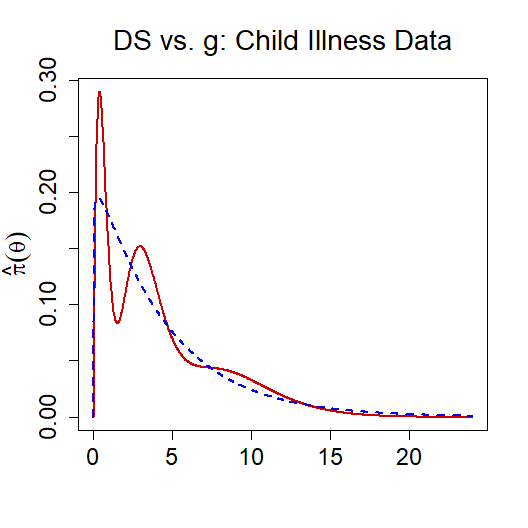### MicroInference

Step 4. The plot shows some very interesting behavior in $$\widehat{\pi}$$. We want to explore the posterior distributions for $$y = 1,3,5,10$$. For those results, we use the microinference functions.

child.micro.1 <- DS.micro.inf(child.ds, y.0 = 1)
child.micro.3 <- DS.micro.inf(child.ds, y.0 = 3)
child.micro.5 <- DS.micro.inf(child.ds, y.0 = 5)
child.micro.10 <- DS.micro.inf(child.ds, y.0 = 10)

By plotting the posterior distributions we see how the distributions change based on the number of times a child is ill. The plots for each of the four microinferences are shown below.

plot(child.micro.1, xlim = c(0,10), main = "y = 1")
plot(child.micro.3, xlim = c(0,10), main = "y = 3")
plot(child.micro.5, xlim = c(0,10), main = "y = 5")
plot(child.micro.10, xlim = c(0,20), main = "y = 10")